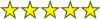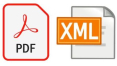# wxMaxima

by Andrej Vodopivec

Operating system: Windows

Publisher: Andrej Vodopivec

Last revision: Last weekwxMaxima is a program which is one of the variants of graphic implementation of Maxima computer algebra system. This system is able to work with numerical and symbolic expressions and is completely free to use, including for commercial purposes. The main benefit of this solution for ordinary users is that it helps to build and solve mathematical formulas and equations. In addition, wxMaxima performs a number of other useful mathematical operations: integration, differentiation, Laplace transformation, numeric series and vectors construction, work with matrices and much more.

The program perfectly "understands" fractions, floating point numbers and contains a large "arsenal" of tools for analytical calculations. The wxMaxima interface is as simple and russified as possible. It consists of a workspace and a toolbar that you can use to build expressions, charts, lists, tensors, and the like. Included with wxMaxima you will find all the necessary documentation and reference materials (partially translated) to help you understand the features of this software solution.

- is a very convenient graphic shell of Maxima's computer algebra system;

- is used to build and calculate symbolic and numeric expressions;

- works with matrices, vectors, equations, tensors, graphs;

- performs differentiation, integration, Laplace transformation, row decomposition and so on;

- is accompanied by detailed documentation.